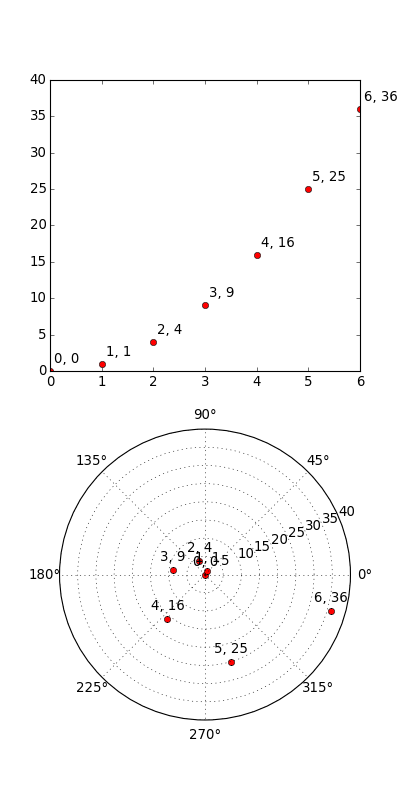#### Previous topic

pylab_examples example code: toggle_images.py

#### Next topic

pylab_examples example code: tricontour_demo.py

# pylab_examples example code: transoffset.py¶```#!/usr/bin/env python

'''
This illustrates the use of transforms.offset_copy to
make a transform that positions a drawing element such as
a text string at a specified offset in screen coordinates
(dots or inches) relative to a location given in any
coordinates.

Every Artist--the mpl class from which classes such as
Text and Line are derived--has a transform that can be
set when the Artist is created, such as by the corresponding
pylab command.  By default this is usually the Axes.transData
transform, going from data units to screen dots.  We can
use the offset_copy function to make a modified copy of
this transform, where the modification consists of an
offset.
'''

import pylab as P
from matplotlib.transforms import offset_copy

X = P.arange(7)
Y = X**2

fig = P.figure(figsize=(5,10))
ax = P.subplot(2,1,1)

# If we want the same offset for each text instance,
# we only need to make one transform.  To get the
# transform argument to offset_copy, we need to make the axes
# first; the subplot command above is one way to do this.

transOffset = offset_copy(ax.transData, fig=fig,
x = 0.05, y=0.10, units='inches')

for x, y in zip(X, Y):
P.plot((x,),(y,), 'ro')
P.text(x, y, '%d, %d' % (int(x),int(y)), transform=transOffset)

# offset_copy works for polar plots also.

ax = P.subplot(2,1,2, polar=True)

transOffset = offset_copy(ax.transData, fig=fig, y = 6, units='dots')

for x, y in zip(X, Y):
P.polar((x,),(y,), 'ro')
P.text(x, y, '%d, %d' % (int(x),int(y)),
transform=transOffset,
horizontalalignment='center',
verticalalignment='bottom')

P.show()
```

Keywords: python, matplotlib, pylab, example, codex (see Search examples)